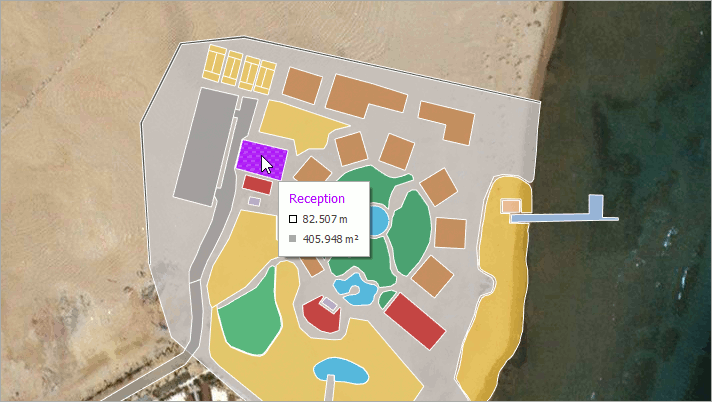# GeoUtils Class

Cartography measurement API for maps with a geographical coordinate system.

Namespace: DevExpress.XtraMap

Assembly: DevExpress.XtraMap.v19.1.dll

## Declaration

``public static class GeoUtils``

## Remarks

The GeoUtils class provides methods that allow you to calculate geometric values (for example, a shape perimeter or area) based on geographical coordinates.The following list contains the main GeoUtils methods:

• CalculateArea(MapShape) computes a map shape’s total area, in square meters.

``````private void mapControl_SelectionChanged(object sender, MapSelectionChangedEventArgs e) {
MapShape mapShape = vectorItemsLayer.SelectedItem as MapShape;
double areaByShape = GeoUtils.CalculateArea(mapShape)/1000000;
label.Text = \$"Shape Area: {areaByShape:F2} km²";
}
``````
• CalculateArea(IList<GeoPoint>) calculates an area of the region given by geographical points, in square meters.

``````double areaByPoints = GeoUtils.CalculateArea(new List<GeoPoint> {
new GeoPoint(41, -109),
new GeoPoint(41, -102),
new GeoPoint(37, -102),
new GeoPoint(37, -109)
});
``````
• CalculateBearing(GeoPoint, GeoPoint) computes an angle between the Geodetic North direction and a line given by two points, clockwise in degrees.

``````double bearing = GeoUtils.CalculateBearing(new GeoPoint(36.1, -115.1), new GeoPoint(35.9, -115.9));
``````
• CalculateCenter(GeoPoint, GeoPoint) finds a center point between two geographical points.

``````GeoPoint centerPoint = GeoUtils.CalculateCenter(
new GeoPoint(35.286, -111.1724),
new GeoPoint(38.3802, -110.9307));
``````
• CalculateDistance(GeoPoint, GeoPoint) determines the distance between two geographical points, in meters.

``````double distance = GeoUtils.CalculateDistance(new GeoPoint(36.1, -115.1), new GeoPoint(34, -118.2));
``````
• CalculateLength(IList<GeoPoint>) calculates the polyline length, in meters.

``````double lineLength = GeoUtils.CalculateLength(new List<GeoPoint> {
new GeoPoint(35.286, -111.1724),
new GeoPoint(38.3802, -110.9307),
new GeoPoint(36.9365, -102.493)
});
``````
• CalculateStrokeLength(MapShape) returns the map shape’s stroke length, in meters.

``````private void mapControl_SelectionChanged(object sender, MapSelectionChangedEventArgs e) {
MapShape mapShape = vectorItemsLayer.SelectedItem as MapShape;
double strokeLength = GeoUtils.CalculateStrokeLength(mapShape);
label.Text = \$"StokeLength: {strokeLength / 1000:F2} km";
}
``````

Object
GeoUtils